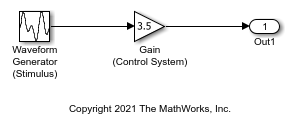# Switch Between Output Waveforms During Code Execution for Waveform Generator Block

This example shows how to generate code that enables you to switch between stimulus waveforms during code execution.

For a Waveform Generator block, you cannot make the parameters of a waveform, such as amplitude and phase shift, tunable in the generated code. Instead, you can generate code that enables you to choose an active waveform from a set of waveform variants that you specify in the block. During execution of the code, you activate a variant by adjusting the value of a global structure field.

You must set the model configuration parameter Default parameter behavior to `Tunable`. Then, by default, block parameters in the model appear tunable in the generated code. These parameters can consume large amounts of memory for a large model.

Create the model `ex_switch_waveform`.

```open_system('ex_switch_waveform') ```In the Waveform Generator block, configure this waveform:

```square(amp,10,0,dutyCycle) ```

In the base workspace, create the variables `amp` and `dutyCycle`.

```amp = 2.71; dutyCycle = 50; ```

This waveform represents the baseline stimulus that you want the application to use at the start of execution.

Suppose that, during execution, you want to observe the effects of changing the waveform frequency from 10 to 15 and the phase shift from 0 to 0.5. In the Waveform Generator block, add these waveform variants:

```square(amp,10,0.5,dutyCycle) square(amp,15,0,dutyCycle) square(amp,15,0.5,dutyCycle) ```

Set the model configuration parameter Default parameter behavior to `Tunable`.

```set_param('ex_switch_waveform','DefaultParameterBehavior','Tunable') ```

Generate code from the model.

```rtwbuild('ex_switch_waveform') ```
```### Starting build procedure for: ex_switch_waveform ### Successful completion of code generation for: ex_switch_waveform ```

The generated file `ex_switch_waveform.h` defines the standard structure type that stores tunable parameter data for the model. The structure contains a field whose value represents the active waveform.

```file = fullfile('ex_switch_waveform_grt_rtw','ex_switch_waveform.h'); rtwdemodbtype(file,'/* Parameters (default storage) */',... '/* Real-time Model Data Structure */',1,0) ```
```/* Parameters (default storage) */ struct P_ex_switch_waveform_T_ { real_T WaveformGeneratorStimulus_Selec; /* Mask Parameter: WaveformGeneratorStimulus_Selec * Referenced by: '<S1>/Switch' */ real_T GainControlSystem_Gain; /* Expression: 3.5 * Referenced by: '<Root>/Gain (Control System)' */ }; ```

The file `ex_switch_waveform_data.c` defines a global structure variable and initializes the field value to `1`. This value represents the baseline waveform.

```file = fullfile('ex_switch_waveform_grt_rtw','ex_switch_waveform_data.c'); rtwdemodbtype(file,'/* Block parameters (default storage) */','};',1,1) ```
```/* Block parameters (default storage) */ P_ex_switch_waveform_T ex_switch_waveform_P = { /* Mask Parameter: WaveformGeneratorStimulus_Selec * Referenced by: '<S1>/Switch' */ 1.0, /* Expression: 3.5 * Referenced by: '<Root>/Gain (Control System)' */ 3.5 }; ```

The file `ex_switch_waveform.c` defines the model execution function. The function uses a `switch` statement to determine the value of the active waveform, and then calculates the value of the root-level Outport block, `Out1`.

```file = fullfile('ex_switch_waveform_grt_rtw','ex_switch_waveform.c'); rtwdemodbtype(file,'switch ((int32_T)',... 'ex_switch_waveform_Y.Out1 =',1,1) ```
``` switch ((int32_T)ex_switch_waveform_P.WaveformGeneratorStimulus_Selec) { case 1: temp = temp - floor(temp) <= 0.5 ? 2.71 : -2.71; break; case 2: temp = temp_0 - floor(temp_0) <= 0.5 ? 2.71 : -2.71; break; case 3: temp = temp_1 - floor(temp_1) <= 0.5 ? 2.71 : -2.71; break; default: temp = temp_2 - floor(temp_2) <= 0.5 ? 2.71 : -2.71; break; } /* End of MultiPortSwitch: '<S1>/MultiportSwitch' */ /* Outport: '<Root>/Out1' incorporates: * Gain: '<Root>/Gain (Control System)' */ ex_switch_waveform_Y.Out1 = ex_switch_waveform_P.GainControlSystem_Gain * temp; ```

During code execution, to change the active waveform, adjust the value of the structure field in the global parameters structure.